# High School Math : Understanding Geometric Sequences

## Example Questions

### Example Question #1 : Geometric Sequences

Which of the following is a geometric sequence?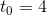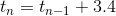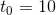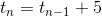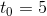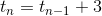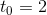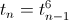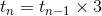Explanation:

A geometric sequence is one in which the next term is found by mutlplying the previous term by a particular constant. Thus, we look for an implicit definition which involves multiplication of the previous term. The only possibility is: